# Angles of Elevation and Depression

Welcome to Class !!

In today’s Mathematics class, We will be discussing Angles of Elevation and Depression. We hope you enjoy the class!

CONTENT:    (i) Horizontal and vertical lines

(ii) Angles of elevation

(iii) Measuring angles of elevation and depression

##### Horizontal and Vertical Lines

Horizontal lines are lines that are parallel to the earth. For example, the surface of a liquid in a container, the floor of a classroom, etc. See the diagram below: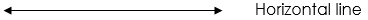Vertical lines are lines that are perpendicular to the horizontal surface, e.g. Wall of a classroom, a swing pendulum, etc.Evaluation:

1. Say whether the following are horizontal or vertical or neither.
• a) A tabletop  b) A door   c) A table leg            d) Top edge of a well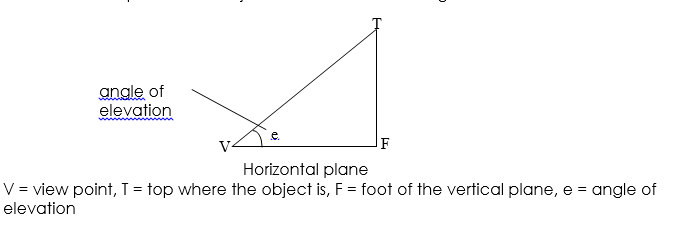NGM BK 2 Chapter 17, pg. 173

Essential Mathematics for JSS BK 2, Chapter 17, pg. 173

##### Angle of Depression

The angle of depression of an object from a given point T is the angle formed when an observer looks down to see an object below his head.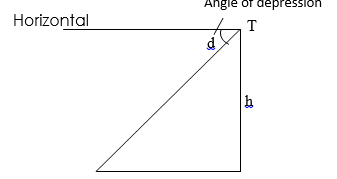Thus the angle of elevation is equal in size to the angle of depression. (Alternate angles are equal i.e. d = e)

NGM BK 2 Chapter 20, pgs. 166 – 167

##### Measuring Angles of Elevation and Depression

When constructing angles of elevation and depression, the use of scale drawing is necessary in order to have effective construction of angle. Consider the diagram below; find the height of the flagpole to the nearest metre using a suitable scale.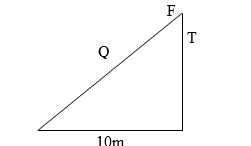Solution

By construction, choose a scale of 1cm to represent 2m.

The height of the flagpole PT = 3cm, converted to m, will give 2 x 3 = 6

Example 2: The angle of elevation of the top of a tower 42m away from a point on the level ground is 36o, find the height of the tower.

SolutionBy construction, using a suitable scale of 1cm represented by 6cm, then PR = = 7cm

The length TR = 5.0cm converting back to metre, we have;

Length TR = 5 x 6 = 30m

Example 3: From the top of a building 20m high, the angle of depression of a car is 45o, find the distance of the car from the foot of the building.

Solution

Rough sketch:T = top of the building, C = car, F = foot of the building

CF is the distance of the car from the foot of the building

Since the angle of depression equal angle of elevation;

By construction, using a suitable scale of 1cm represents 5m

For 20m, we have  = 4cm

Length CF 4cm

By conversion, length CF = 4 x 5 = 20m

EVALUATION

1. A tower PQ is 10m high if the distance from point R to P is 50m on the ground, find the angle of elevation of Q from R1. From the top of a cliff of 200m high, Martins observes that the angle of depression of a boat at sea is 35o, find the distance between the boat and the foot of the cliff.

GENERAL EVALUATION

1. A boat is 180m from the foot of a vertical cliff of height 80m. find by scale drawing the angle of depression of the boat measured from the top of the cliff.
2. A boy is flying a kite. The string is 25m long and is at an angle of 42o to the horizontal. Using a scale diagram, find how high the kite is above the boy’s head?

REVISION QUESTION

1. The angle of elevation of point P from point Q is 40o. PQ = 45km. How high is point P above the level of point Q?
2. A girl with an eye-level height of 1.65m observes that the angle of elevation of the top of the tower 20m away is 40o. Calculate the height of the tower.

NGM BK 2 chapter 20, page 166 – 169

Essential mathematics for JSS BK 2, chapter 23, pg. 295 – 297

Exercise 23.1 No 1, 2 & 3 page 296

WEEKEND ASSIGNMENT

1. Calculate the size of the fourth angle if three angles of a quadrilateral are 65o, 115o and 125o respectively A. 35o     B. 55o     C. 45o    D. 75o
2. Calculate the number of side of a regular polygon whose total angles is 1080o  A. 4     B. 6      C. 8     D. 10
3. PRQS is a rectangle with the side 3cm and 4cm, if its diagonal cross at O, calculate the length of PO.          A. 3.5cm     B. 5.0cm      C. 2.5cm      D. 4.0cm
4. If the angle of a quadrilateral could be x, 2x, 4x and 5x respectively, what would be the value of x?      A. 60o     B. 90o      C. 15o     D. 30o
5. If the angle of elevation of a building from a point on the ground is 43o. What is the angle of depression? A. 47o    C. 53o    C. 43o     D. 32o

THEORY

1. From the top of a building 50m high, the angle of depression of a car is 55o, find the distance of the car from the foot of the building.
2. Find the height of the flagpole in the diagram below to the nearest metre.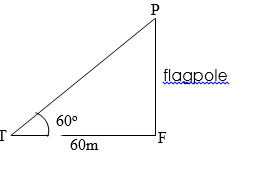We have come to the end of this class. We do hope you enjoyed the class?

Should you have any further question, feel free to ask in the comment section below and trust us to respond as soon as possible.

In our next class, we will be talking about Bearing and Distances. We are very much eager to meet you there.

Are you a Parent? Share your quick opinion and win free 2-month Premium Subscription

### 1 thought on “Angles of Elevation and Depression ”

1.new general mathematics2, exercise 20b numbers 1ans 2 . please explain 5his to me

Don`t copy text!